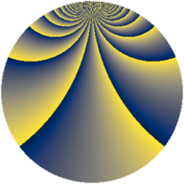# Properties

 Label 1815.2.buLevel $1815$ Weight $2$ Character orbit 1815.bu Rep. character $\chi_{1815}(38,\cdot)$ Character field $\Q(\zeta_{220})$ Dimension $20800$ Sturm bound $528$

# Related objects

## Defining parameters

 Level: $$N$$ $$=$$ $$1815 = 3 \cdot 5 \cdot 11^{2}$$ Weight: $$k$$ $$=$$ $$2$$ Character orbit: $$[\chi]$$ $$=$$ 1815.bu (of order $$220$$ and degree $$80$$) Character conductor: $$\operatorname{cond}(\chi)$$ $$=$$ $$1815$$ Character field: $$\Q(\zeta_{220})$$ Sturm bound: $$528$$

## Dimensions

The following table gives the dimensions of various subspaces of $$M_{2}(1815, [\chi])$$.

Total New Old
Modular forms 21440 21440 0
Cusp forms 20800 20800 0
Eisenstein series 640 640 0

## Trace form

 $$20800q - 60q^{3} - 164q^{6} - 156q^{7} + O(q^{10})$$ $$20800q - 60q^{3} - 164q^{6} - 156q^{7} - 92q^{10} - 118q^{12} - 128q^{13} - 82q^{15} - 824q^{16} - 46q^{18} - 152q^{21} - 244q^{22} - 140q^{25} - 60q^{27} - 184q^{28} - 62q^{30} - 344q^{31} - 192q^{33} - 228q^{36} - 180q^{37} - 184q^{40} - 96q^{42} - 176q^{43} + 50q^{45} - 352q^{46} - 36q^{48} - 148q^{51} - 188q^{52} - 8q^{55} - 184q^{57} - 264q^{58} - 90q^{60} - 352q^{61} - 118q^{63} - 156q^{66} - 192q^{67} - 288q^{70} - 4q^{72} - 96q^{73} - 34q^{75} - 176q^{76} - 174q^{78} - 164q^{81} - 172q^{82} - 164q^{85} + 14q^{87} + 100q^{88} - 302q^{90} - 744q^{91} - 32q^{93} - 328q^{96} - 92q^{97} + O(q^{100})$$

## Decomposition of $$S_{2}^{\mathrm{new}}(1815, [\chi])$$ into newform subspaces

The newforms in this space have not yet been added to the LMFDB.